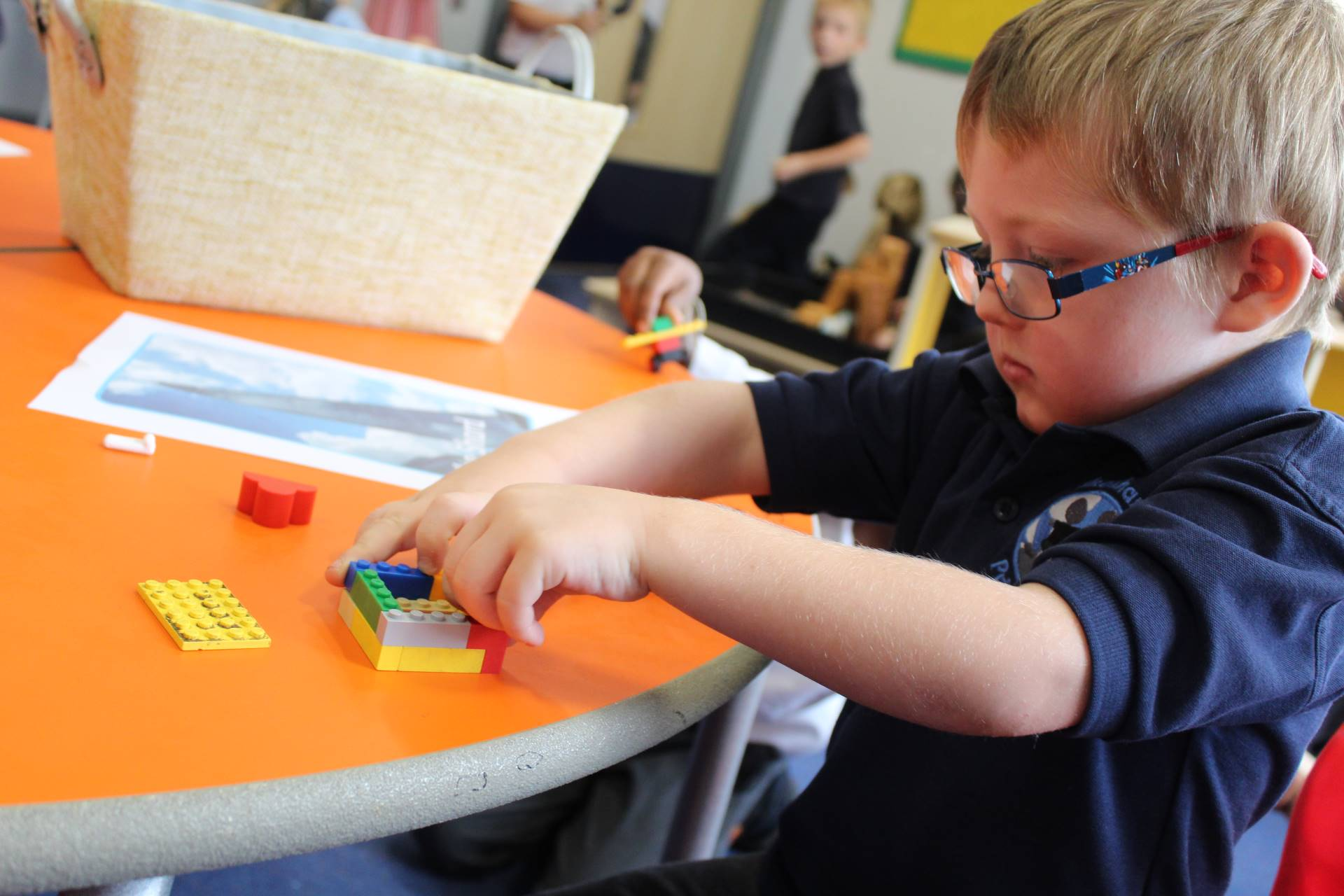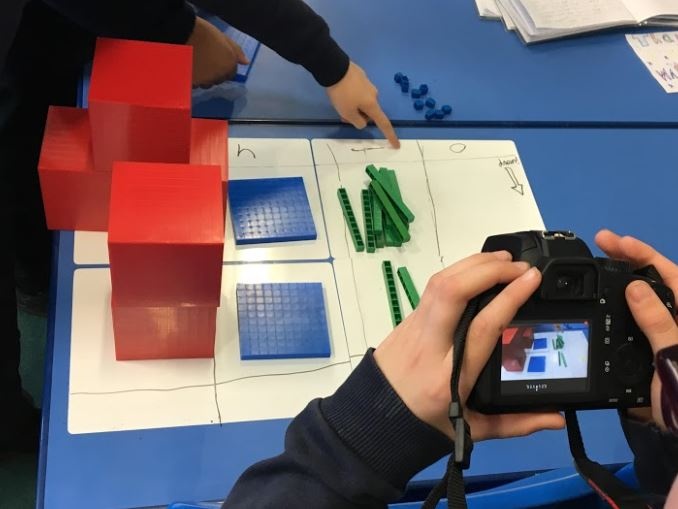Quick Links

Useful Links# Mathematics

Children are taught to be confident in every area of mathematics. They will develop and extend their problem-solving capabilities through a wide variety of mathematical contexts. We work within the National Curriculum framework, which includes using and applying mathematics, number and algebra, shape, space and measures and handling data. We also use a wide variety of computer software to support children’s mathematical understanding. We believe that children learn by doing real mathematics and have lots of practical activities to ensure that children gain a deep understanding of mathematical concepts. We start with teaching the concept, children get to practice and then apply to problem solving.

SSIF project

Movies